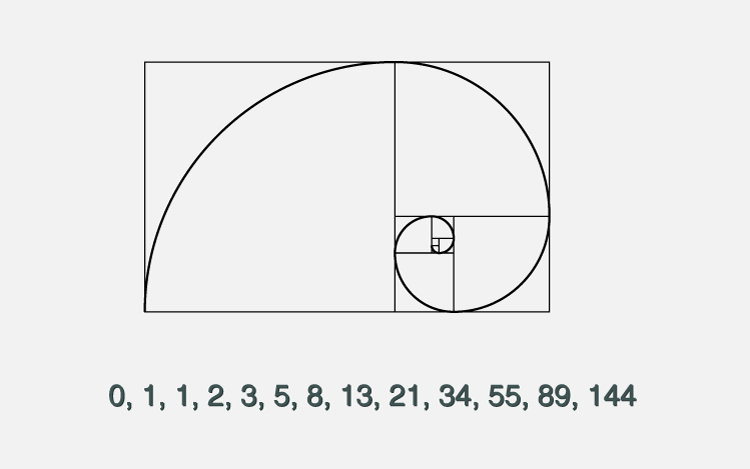# C++ Program to Display Fibonacci Series

In this article, you will learn to print fibonacci series in C++ programming (up to nth term, and up to a certain number).To understand this example, you should have the knowledge of the following C++ programming topics:

The Fibonacci sequence is a series where the next term is the sum of pervious two terms. The first two terms of the Fibonacci sequence is 0 followed by 1.

`The Fibonacci sequence: 0, 1, 1, 2, 3, 5, 8, 13, 21`

## Example 1: Fibonacci Series up to n number of terms

``````#include <iostream>
using namespace std;

int main()
{
int n, t1 = 0, t2 = 1, nextTerm = 0;

cout << "Enter the number of terms: ";
cin >> n;

cout << "Fibonacci Series: ";

for (int i = 1; i <= n; ++i)
{
// Prints the first two terms.
if(i == 1)
{
cout << " " << t1;
continue;
}
if(i == 2)
{
cout << t2 << " ";
continue;
}
nextTerm = t1 + t2;
t1 = t2;
t2 = nextTerm;

cout << nextTerm << " ";
}
return 0;
}
``````

Output

```Enter the number of terms: 10
Fibonacci Series: 0, 1, 1, 2, 3, 5, 8, 13, 21, 34, ```

## Example 2: Program to Generate Fibonacci Sequence Up to a Certain Number

``````#include <iostream>
using namespace std;

int main()
{
int t1 = 0, t2 = 1, nextTerm = 0, n;

cout << "Enter a positive number: ";
cin >> n;

// displays the first two terms which is always 0 and 1
cout << "Fibonacci Series: " << t1 << ", " << t2 << ", ";

nextTerm = t1 + t2;

while(nextTerm <= n)
{
cout << nextTerm << ", ";
t1 = t2;
t2 = nextTerm;
nextTerm = t1 + t2;
}
return 0;
}
``````

Output

```Enter a positive integer: 100
Fibonacci Series: 0, 1, 1, 2, 3, 5, 8, 13, 21, 34, 55, 89, ```